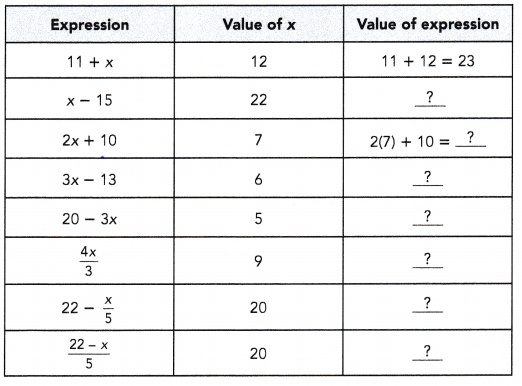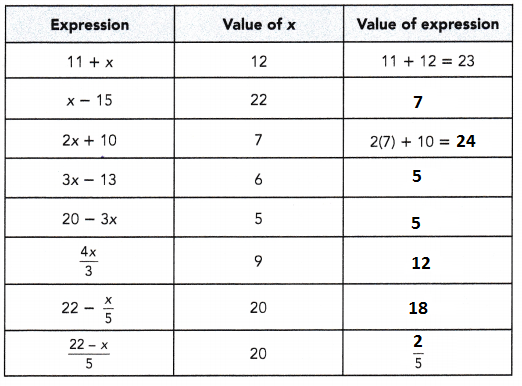# Math in Focus Grade 6 Chapter 7 Lesson 7.2 Answer Key Evaluating Algebraic Expressions

Practice the problems of Math in Focus Grade 6 Workbook Answer Key Chapter 7 Lesson 7.2 Evaluating Algebraic Expressions to score better marks in the exam.

## Math in Focus Grade 6 Course 1 A Chapter 7 Lesson 7.2 Answer Key Evaluating Algebraic Expressions

### Math in Focus Grade 6 Chapter 7 Lesson 7.2 Guided Practice Answer Key

Evaluate each algebraic expression for the given value of x.

Question 1.Explanation:
An expression is a term in that describes a group of variables, numbers and operators.
Operators include division, multiplication, addition and subtraction.
Variables in expression are usually denoted as x and y, but it can be and other symbol.
The expressions can be written as verbal phrase or algebraic expression.

### Math in Focus Course 1A Practice 7.2 Answer Key

Evaluate each expression for the given value of the x.

Question 1.
x + x + 5 when x = 7
19
Explanation:
x = 7
by substituting x value as 7
x + x + 5 = 7 + 7 + 5 = 19

Question 2.
3x + 5 when x = 5
20
Explanation:
3x + 5, where as
x = 5
by substituting x value =5
3 x 5 + 5 = 15 + 5 = 20

Question 3.
5y – 8 when y = 3
7
Explanation:
5 x y – 8
y = 3
by substituting y value = 3
5 x 3 – 8
15 – 8 = 7

Question 4.
40 – 9y when y = 2
22
Explanation:
40 – 9y
y = 2
by substituting y value = 2
40 – 9 x 2 = 40 – 18 = 22

Question 5.
33 – 7w + 6 when w = 4
19
Explanation:
33 – 7w + 6
w = 4
by substituting w value = 4
33 – 7 x 4 = 33 – 14 = 19

Question 6.
$$\frac{7w}{2}$$ when w = 18
63
Explanation:
= $$\frac{7w}{2}$$
w = 18
by substituting w value = 18
= $$\frac{7 x 18}{2}$$
= $$\frac{126}{2}$$ = 63

Question 7.
4 + $$\frac{5z}{6}$$ when z = 12
14
Explanation:
4 + $$\frac{5z}{6}$$
z = 12
by substituting z value = 12
= 4 + $$\frac{5×12}{6}$$
= 4 + $$\frac{60}{6}$$
= 4+10 = 14

Question 8.
$$\frac{4+5z}{2}$$ when z = 12
32
Explanation:
$$\frac{4+5z}{2}$$
z = 12
by substituting z value =12
= $$\frac{4+5 x 12}{2}$$
= $$\frac{4+60}{2}$$
= $$\frac{64}{2}$$ = 32

Question 9.
20 – $$\frac{4r}{5}$$ when r = 10
16
Explanation:
20 – $$\frac{4r}{5}$$
r = 10
by substituting r value =10
= 20 – $$\frac{4x 10}{5}$$
= 20 – $$\frac{40}{5}$$
= 20 – 8 = 16

Question 10.
$$\frac{8r}{9}$$ – 15 when r = 27
9
Explanation:
$$\frac{8r}{9}$$ – 15
r = 27
by substituting r value =27
= $$\frac{8 x 27}{9}$$ – 15
= $$\frac{216}{9}$$ – 15
24 – 15 = 9

Question 11.
16 – $$\frac{2 z-4}{3}$$ when z = 18
$$\frac{16}{3}$$
Explanation:
16 – $$\frac{2 z-4}{3}$$ when z = 18
by substituting z value = 18
16 – $$\frac{2 z-4}{3}$$
=16 – $$\frac{2 x 18 – 4}{3}$$
=16 – $$\frac{36-4}{3}$$
=16 – $$\frac{32}{3}$$
=$$\frac{48-32}{3}$$
$$\frac{16}{3}$$

Question 12.
16 – $$\frac{2 z}{3}$$ – 4 when z = 18
0
Explanation:
16 – $$\frac{2 z}{3}$$ – 4 when z = 18
by substituting  z value = 18
16 – $$\frac{2 x 18}{3}$$ – 4
= 16 – $$\frac{36}{3}$$ – 4
=16 – 12 – 4
=0

Evaluate each expression when x = 3.

Question 13.
$$\frac{x+1}{2}$$ + $$\frac{5 x-3}{10}$$
$$\frac{16}{5}$$
Explanation:
by substituting  x value = 3 in the given algebraic equation
$$\frac{x+1}{2}$$ + $$\frac{5 x-3}{10}$$
= $$\frac{3+1}{2}$$ + $$\frac{5 x 3 – 3}{10}$$
= $$\frac{4}{2}$$ + $$\frac{12}{10}$$
= 2 + $$\frac{6}{5}$$
= $$\frac{10 + 6}{5}$$
= $$\frac{16}{5}$$

Question 14.
$$\frac{11+x}{2}$$ – $$\frac{9 x-3}{4}$$
1
Explanation:
by substituting  x value = 3 in the given algebraic equation
$$\frac{11+x}{2}$$ – $$\frac{9 x-3}{4}$$
= $$\frac{11+3}{2}$$ – $$\frac{9 x 3-3}{4}$$
= $$\frac{14}{2}$$ – $$\frac{27-3}{4}$$
= $$\frac{7}{1}$$ – $$\frac{24}{4}$$
7 – 6 = 1

Question 15.
$$\frac{7 x-6}{3}$$ + 4(8 + 2x)
61
Explanation:
by substituting  x value = 3 in the given algebraic equation
$$\frac{7 x-6}{3}$$ + 4(8 + 2x)
= $$\frac{7 x 3 -6}{3}$$ + 4(8 + 2 x 3)
= $$\frac{21- 6}{3}$$ + 4(8 + 6)
= $$\frac{15}{3}$$ + 56
= 5 + 56
= 61

Question 16.
13(11 – 3x) – $$\frac{5(16-4 x)}{2}$$
16
Explanation:
by substituting  x value = 3 in the given algebraic equation
13(11 – 3x) – $$\frac{5(16-4 x)}{2}$$
13(11 – 3 x 3) – $$\frac{5(16-4 x 3)}{2}$$
13(11 – 9) – $$\frac{5(16 – 12)}{2}$$
13(2) – $$\frac{5(4)}{2}$$
26 – $$\frac{20}{2}$$
= 26 – $$\frac{20}{2}$$
= 26 – 10
= 16

Question 17.
5(x + 2) + 2(6 – x) + $$\frac{2 x+3}{3}$$
34
Explanation:
by substituting  x value = 3 in the given algebraic equation
5(x + 2) + 2(6 – x) + $$\frac{2 x+3}{3}$$
5(3 + 2) + 2(6 – 3) + $$\frac{2 x 3+3}{3}$$
5(5) + 2(3) + $$\frac{6+3}{3}$$
25 + 6 + 3 = 34

Question 18.
$$\frac{5 x-3}{4}$$ + $$\frac{5(x+5)}{8}$$ + 3(13 – 2x)
29
Explanation:
by substituting  x value = 3 in the given algebraic equation
$$\frac{5 x-3}{4}$$ + $$\frac{5(x+5)}{8}$$ + 3(13 – 2x)
= $$\frac{5 x 3 – 3}{4}$$ + $$\frac{5(3+5)}{8}$$ + 3(13 – 2 x 3)
= $$\frac{15-3}{4}$$ + $$\frac{5(8)}{8}$$ + 3(13 – 6)
= $$\frac{12}{4}$$ + $$\frac{40}{8}$$ + 3(7)
= 3 + 5 + 21
= 29

Question 19.
$$\frac{2 x+4}{5}$$ – $$\frac{x+1}{4}$$ + $$\frac{x}{6}$$
$$\frac{3}{2}$$
Explanation:
by substituting  x value = 3 in the given algebraic equation
$$\frac{2 x+4}{5}$$ – $$\frac{x+1}{4}$$ + $$\frac{x}{6}$$
= $$\frac{2 x 3+4}{5}$$ – $$\frac{3+1}{4}$$ + $$\frac{3}{6}$$
= $$\frac{10}{5}$$ – $$\frac{4}{4}$$ + $$\frac{1}{2}$$
= 2-1+$$\frac{1}{2}$$
=1+$$\frac{1}{2}$$
= $$\frac{3}{2}$$

Question 20.
7x – $$\frac{x}{5}$$ + $$\frac{7-x}{9}$$
21 – $$\frac{3}{5}$$ + $$\frac{4}{9}$$
Explanation:
by substituting  x value = 3 in the given algebraic equation
7x – $$\frac{x}{5}$$ + $$\frac{7-x}{9}$$
= 7 x 3 – $$\frac{3}{5}$$ + $$\frac{7-3}{9}$$
= 21 – $$\frac{3}{5}$$ + $$\frac{4}{9}$$
= 21 – $$\frac{3}{5}$$ + $$\frac{4}{9}$$

Evaluate each of the following when y = 7.

Question 21.
(5y + 2) minus (2y + 5).
18
Explanation:
by substituting  y value = 7 in the given algebraic equation
(5y + 2) minus (2y + 5)
= (5 x 7 + 2) – (2 x 7 + 5).
= (35 + 2) – (14 + 5).
= 37 – 19
= 18

Question 22.
The sum of $$\frac{y}{3}$$ and $$\frac{4y}{9}$$
$$\frac{49}{9}$$
Explanation:
by substituting  y value = 7 in the given algebraic equation
$$\frac{y}{3}$$ + $$\frac{4y}{9}$$
= $$\frac{7}{3}$$ + $$\frac{4 x 7}{9}$$
= $$\frac{7}{3}$$ + $$\frac{28}{9}$$
= $$\frac{21+28}{9}$$
= $$\frac{49}{9}$$

Question 23.
The product of (y + 1)and(y – 1)
$$\frac{4}{3}$$
Explanation:
by substituting  y value = 7 in the given algebraic equation
(y + 1)and(y – 1)
= (7 + 1)and(7 – 1)
= (8)and(6)
= $$\frac{8}{6}$$
= $$\frac{4}{3}$$

Question 24.
8(2y – 1) minus $$\frac{14 y+37}{5}$$
8(2y – 1) minus $$\frac{14 y+37}{5}$$
Explanation:
by substituting  y value = 7 in the given algebraic equation
8(2y – 1) minus $$\frac{14 y+37}{5}$$
= 8(2 X 7 – 1) minus $$\frac{14 X 7 + 37}{5}$$
= 8(2y – 1) minus $$\frac{14 y+37}{5}$$

Question 25.
The quotient of 9(7y – 15) and $$\frac{110-6 y}{4}$$
18
Explanation:
by substituting  y value = 7 in the given algebraic equation
9(7y – 15) and $$\frac{110-6 y}{4}$$
= 9(7 x 7 – 15) and $$\frac{110 – 6 x 7}{4}$$
= 9(49 – 15) and $$\frac{110-42}{4}$$
= 9(34) and $$\frac{68}{4}$$
= 306/17 = 18

Question 26.
The sum of $$\frac{5 y}{6}$$ and 4($$\frac{3 y}{7}$$ + 2y)
$$\frac{443}{6}$$
Explanation:
by substituting  y value = 7 in the given algebraic equation
$$\frac{5 y}{6}$$ and 4($$\frac{3 y}{7}$$ + 2y)
= $$\frac{5 x 7}{6}$$ and 4($$\frac{3 x 7}{7}$$ + 2 x 7)
= $$\frac{35}{6}$$ and 4($$\frac{21}{7}$$ + 14)
= $$\frac{35}{6}$$ and 4(3 + 14)
= $$\frac{35}{6}$$ +68
= $$\frac{35 + 408 }{6}$$
= $$\frac{443}{6}$$

Question 27.
The quotient of ($$\frac{y}{2}+\frac{2 y}{3}$$) and ($$\frac{5 y}{6}-\frac{y}{3}$$)
$$\frac{7}{3}$$
($$\frac{y}{2}+\frac{2 y}{3}$$) and ($$\frac{5 y}{6}-\frac{y}{3}$$)
= ($$\frac{7}{2}+\frac{2 x 7}{3}$$) and ($$\frac{5 x 7}{6}-\frac{7}{3}$$)
= ($$\frac{7}{2}+\frac{14}{3}$$) and ($$\frac{35}{6}-\frac{7}{3}$$)
= ($$\frac{21 + 28}{6}$$) and ($$\frac{35 – 14}{6}$$)
= ($$\frac{49}{6}$$) and ($$\frac{21}{6}$$)
= ($$\frac{49}{6}$$) X ($$\frac{6}{21}$$)
= $$\frac{49}{21}$$
= $$\frac{7}{3}$$